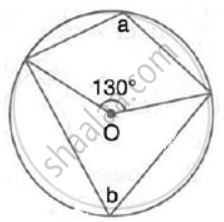Share

# In the Following Figures, O is the Centre of the Circle. Find the Values of A, B and C. - Mathematics

Course

#### Question

In the following figures, O is the centre of the circle. Find the values of a, b and c.#### SolutionHere, b=1/2xx 130°

(Angle ate he centre is double the angle at the circumference subtended by the same chord)

⇒ b = 65°

Now, a + b 180°

(Opposite angles of a cyclic quadrilateral are supplementary)

⇒ a = 180°- 65°= 115°

Is there an error in this question or solution?